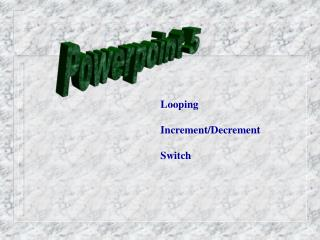DownloadDownload PresentationPowerpoint 5

# Powerpoint 5

Download Presentation## Powerpoint 5

- - - - - - - - - - - - - - - - - - - - - - - - - - - E N D - - - - - - - - - - - - - - - - - - - - - - - - - - -
##### Presentation Transcript

1. Powerpoint 5 Looping Increment/Decrement Switch

2. Flow of Control Iteration/Switch Statements

3. Iteration Loop: A portion of a program that repeats itself a number of times Body: Repeated group of statements Iteration: Each repetition

4. Iteration While (logical expression) { statement(s); }

5. Iteration do { statement(s); } while (logical expression);

6. While (expression) . . logical expression is checked before loop execution Do . while (expression); Logical expression is checked after loop execution Iteration

7. Iteration With a while loop: - Always give a value to a control variable in two places o prior to entering the loop o last statement in the loop With a do/while loop: - Always give a value to a control variable in one place o last statement in the loop

8. Example 1 which_player=-1; //which_player is control variable while (which_player < 0) { cout<<“Enter the following: “; cout<<“**********************”<<endl; cout<<setw(10)<<“1: “<<lname1<<“,”<<fname1<<endl; cout<<setw(10)<<“2: ”<< lname2<<“,”<<fname2<<endl; which_player=thisclass. read_convert_to_int (); if(which_player < 1 || which_player >2) { which_player = -1; } }

9. Example 2 do { cout<<“Enter the following: “; cout<<“**********************”<<endl; cout<<setw(10)<<“1: “<<lname1<<“,”<<fname1<<endl; cout<<setw(10)<<“2: ”<< lname2<<“,”<<fname2<<endl; which_player=thisclass. read_convert_to_int (); if(which_player < 1 || which_player >2) { which_player = -1; } while (which_player == -1) }

10. Increment/Decrement Operators • A variable may be incremented/decremented using a shortcut • The increment/decrement takes place before or after the actual operation on a variable dependending upon placement of the operator

11. Increment/Decrement Operators • postincrement: when the operator is physically after the variable to be incremented • k = i ++; • if i were = 3; k would be 3 • preincrement: when the operator is physically before the variable to be incremented • k = ++i; • if i were = 3; k would be 4

12. Increment/Decrement Operators • postdecrement: when the operator is physically after the variable to be incremented • k = i --; • if i were = 3; k would be 3 • predecrement: when the operator is physically before the variable to be incremented • k = - - i; • if i were = 3; k would be 2

13. Increment/Decrement equivalent k = k + I; k = k * 4; K = k - y; k = k / (y + w + z); k = k % 13; operation k += I; k *=4; k -=y; k /= y + w + z; k % = 13;

14. Self Test, pages 394-95 int count = 3; while (count -- > 0) { cout<<count<<“ “; int count = 3; while (-- count > 0) { cout<<count<<“ “;

15. Iteration for (init exp; test; increment) { statement(s); };

16. Iteration • For loop • Initializing variable/number/ expression;declarations are permissable • if test expression is true, statements are executed • increment/decrement counter one or more

17. For Loop Continued • for (<init-exp>;<test>;<increment/decrement) • { • stmt(s) • } • Initializing expression, is executed • Expression evaluated for true/false; terminates if false • Otherwise statements are executed • Increment/decrement is executed

18. For Loop Class Exercise Write a class function to: 1) input n variables, 2) sum each into a field called total The number, n is passed to the function as an argument. The function definition is: void abc::getgrades (int cnt)

19. For Loop Class Exercise void abc::getgrades (int cnt) { cout<<“Enter a Grade: “<<endl; for (int k=1;k<cnt;k++) { x= thisclass. read_convert_to_int (); while(x < 1|| x > 2) { cout<<“Input error. Try Again!”<,endl; x= thisclass. read_convert_to_int (); } }

20. For or While?? • Use a for loop when there is a numerical calculation changed by an equal amount each iteration • if circumstances are such that you want the loop to be executed at least one time, use the do-while • if it is possible that the loop be skipped sometimes, use the while-loop

21. Switch Statement

22. switch (control expression) { case constant: statement(s) break; case constant: statement(s) break; . . default: statement(s) }

23. 1. When statement is executed, one of a number of branches is determined by the control statement. 2. The control statement is in () after the switch 3. Note preferred indenting pattern 4. Control statement must always return a char or an integer

24. 1. Upon execution, the control statement is evaluated 2. The computer then looks at the values after each case until it finds a match, I.e. constant equal to the return and executes the code until a “break” statement is encountered! 3. Note that you may not have more than one occurance of any constant

25. Let’s Practice • Write a sequence of code that will access a function, based upon the constant within a switch statement • Assume that an “a” means to call a function, addit, that receives two integer values - x and y • An “m” means to call a function, multit, that receives two integer values - x and y • A “d” means to call a function, divit, that again receives two integer values - x and y • A “r” means to call a function, modit, that again receives the same two values • If the constant is not recognized, it is an error